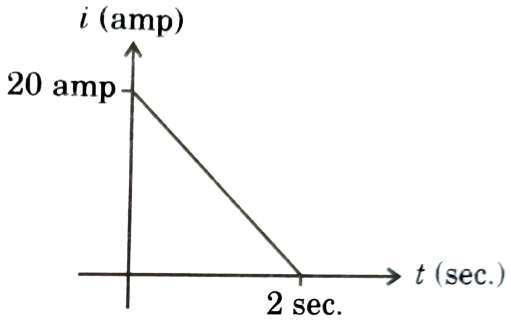Magnetic flux and induced emf
Question

# Due to change in magnetic flux linked with a coil of resistance 10 ohm, a current is induced in it. The variation of induced current i in amperes) with time t (in seconds) is shown in figure. The magnitude of change in flux through the coil (from t = 0 to t = 2 seconds) in Webers is:Moderate
Solution

## Change in flux $\mathrm{\Delta \varphi }=\mathrm{\Delta QR}$Where $\mathrm{\Delta Q}$ is charge that has passed through loop and R is loop resistance.

Get Instant Solutions